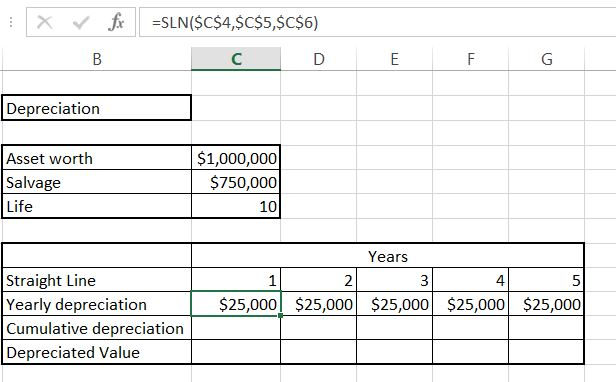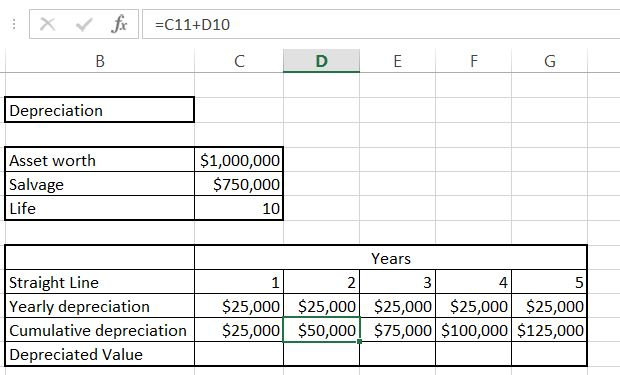### How To Calculate Straight Line Depreciation

In this Excel lesson you will learn yourself how to calculate Straight Line Depreciation.

The asset:

• it is 1 million of dollars worth
• salvage value is \$750 000
• life of the asset is 10 yearsTo calculate straight line depreciation we will use SLN Excel formula.

Syntax of Straight Line Depreciation formula is:

• cost
• salvage
• life

Luckily we already have all data we need.

To calculate yearly depreciation use

=SLN(\$C\$4,\$C\$5,\$C\$6) formula.Absolute reference is needed because the data will not change in the table. For every year it is the same amount of \$25 000 of yearly cost.

Cumulative depreciation is just =C11+D10. Drag it right to calculate all of them. This is just current year value plus sum of previous ones.Deprecated value is =\$C\$4-C11 which is the starting value reduced by cumulative depreciation.This is Straight Line Depreciation formula and the easiest way to calculate Straight Line Depreciation in Excel.The difference between straight line depreciation and declining balance is that for straight line depreciation yearly depreciation is the same value and for declining balance it is different (based on percentage).

## Template

```Further reading: